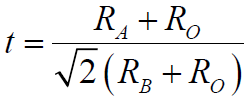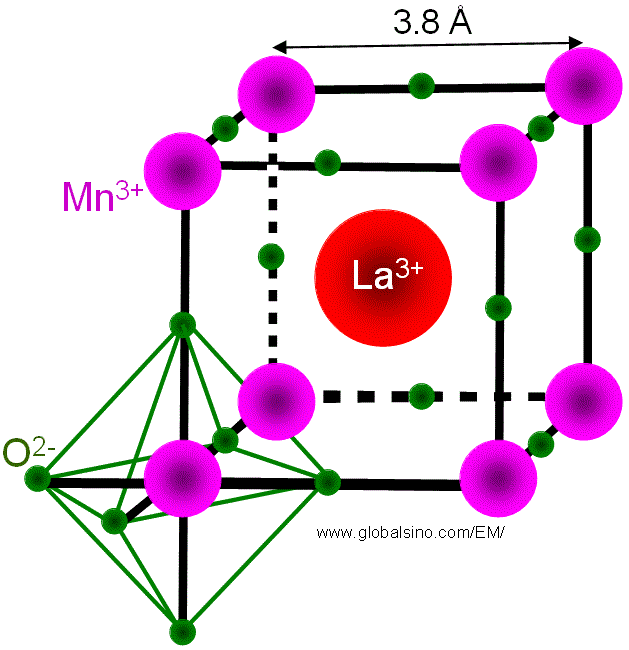Perovskite Structures
- Practical Electron Microscopy and Database -
- An Online Book -

http://www.globalsino.com/EM/

=================================================================================

The name “perovskite” originally refers to a type of minerals with chemical formula CaTiO3, named after Russian mineralogist L. A. Perovski. In general, any crystals with the same structure as CaTiO3 are categorized into the perovskite structure.

Most perovskite crystals are oxides with the general formula ABO3, where A and B represent two different cations and O represents oxygen elements. The A-site cations are larger than B-site cations. Perovskite oxides (ABO3) can be described as the framework of corner-shared BO6 octahedra with 12-coordinated A cations. The unit cell of an ideal perovskite structure is a cube, where the A-site cations locate at the corners of the cube, the B-site cation sits in the body center and oxygen sits in the face centers as shown in Figure 3533a. The structure is a corner-linked oxygen octahedral network. The octahedra are linked in a regular cubic array, resulting in high-symmetry cubic m-3m prototype structure. The 6-fold-coordinated B-site in the center of the oxygen octahedron is occupied by a small highly charged cation with a valence state of 3+, 4+, 5+ or 6+, and the larger 12-fold coordinated A-site between octahedra is occupied by a larger cation with a valence state of 1+, 2+, or 3+.

The main advantage of the perovskite structures is the large flexibility in tailoring the chemical composition and lattice parameter(s) of the system by substituting the different cations that present on both the A and B sites without changing the overall structure completely.Figure 3533a. Schematic illustration of ideal perovskite structure.

However, most perovskite structures do not have such ideal cubic symmetry and are normally distorted, even for the prototype perovskite, CaTiO3. For instance, the properties of the cations on the A-sites and on the B-sites often induce common distortions such as cation displacements within the octahedra and tilting of the octahedra. In general, the degree of distortion in ABO3 perovskites can be determined by ,----------------------------- 

where,
RA, RB and RO -- The ionic radii of the A-site cation, B-site cation and oxygen anion, respectively.
t -- Goldschmidt tolerance factor.

In an ideal cubic perovskite crystalline structure the A-site and B-site cations optimize their equilibrium bond distances to the oxygen elements without inducing any distortion of the unit cell at t = 1. When 0.75 < t < 1.05 (that almost all the perovskites have), a distorted perovskite structure can normally be stabilized. Note that most of the cubic perovskites have t values in between 0.8 and 0.9.

All the ferroelectric materials today are based on corner-linked oxygen octahedral structures. The simplest configuration is the well-known perovskite structure. In EELS, perovskite type ferroelectric and high-k dielectric materials, such as BaTiO3 and SrTiO3, normally show only one interband plasmon peak [2–5].

For perovskite cell structures, octahedral oxygen (O) bonding around the atom (Mn for LaMnO3 as shown in Figure 3533b) in B-site generates the crystal field around the B-site atom. The valence states of the atoms (e.g. Mn) are fixed by charge neutrality. In the case of LaMnO3, if the parent compound is doped with Sr2+ for La3+, then Mn3+ (d3) is replaced by Mn4+ in the lattice.Figure 3533b. Octahedral oxygen bonding around the Mn atom in LaMnO3.

Table 3533a. Atomistic positions in cubic perovskites.

 Site Location Co-ordinates A cation (2a) (0, 0, 0) B cation (2a) (1/2 , 1/2 , 1/2) O anion (6b) (1/2 , 1/2 , 0) (1/2 , 0, 1/2) (0, 1/2 , 1/2 )

Table 3533b. Atomistic positions in orthorhombic perovskites.

 Site Location Co-ordinates A cation (4c) ±[(u, v, 1/4)(1/2-u, v+1/2, 1/4)] B cation (4b) (1/2, 0, 0) (1/2, 1/2, 0) (0, 0, 1/2) (0, 1/2, 1/2) O(1) anion (4c) ±[(m, n, 1/4) (1/2-m, n+1/2, 1/4)] O(2) anion (8d) ± [(x, y, z) (1/2-x, y+1/2, 1/2-z) (-x, -y, z+1/2) (x+1/2, 1/2-y, -z)]
 * u, v, m, n are dependent on the particular structure under consideration.

Table 3533c. Atomic positions for rhombohedral perovskites.

 Site Location Co-ordinates A cation (6a) (0, 0, 1/4) B cation (6b) (0, 0, 0) O anion (18e) (x, 0, 1/4)
 * The co-ordinates are based on hexagonal axes.

Table 3533d. Atomic positions for hexagonal perovskites.

 Site Location Co-ordinates A cation 2a (0, 0, z) A cation 4b (1/3 , 2/3 , z) B cation 6c (x, 0, z) O(1) anion 6c (x, 0, z) O(2) anion 6c (x, 0, z) O(3) anion 2a (0, 0, z) O(4) anion 4b (1/3 , 2/3 , z)

Table 3533e. Examples of perovskite crystals.

 Substance A-site B-site Mobile ions Space Group Crystal structure Lattice constant (nm) Angles Ionic conductivity/Scm-1 (at °C) Remarks Ag3SI Ag+ 1x10-2 at 25 No-oxide anti-perovskite-type Ba0.5Sr0.5Co0.8Fe0.2O3-δ (BSCF) Ba & Sr Co & Fe Good catalytic activity above 600 °C BaTiO3 0.401 BiFeO3 R3CH Rhombohedral a = 0.55775; b = 0.55775; c = 1.38616 90, 90, 120 CaCu3Ti4O12 Ca2+ & Cu2+ High permittivity: good for capacitors CaSnO3 0.392 CaTiO3 0.384 CaZrO3 0.402 CeAlO3 P4/mmm a = 0.37669; c = 0.37967 CeCrO3 Pm-3m 0.389 CeFeO3 Rhombohedral 0.39 90, 90, 90 CeGaO3 0.3879 CeVO3 Pbnm a = 0.5514; b = 0.5557; c = 0.7808 CrBiO3 Tetragonal a = 0.777; c = 0.808 90, 90, 90 CsCdBr3 0.533 CsHgBr3 0.577 CsHgCl3 0.544 CsIO3 0.466 CsPbCl3 Cl- 1.2x10-3 at 500 No-oxide perovskite CsPbBr3 Br- 8x10-4 at 500 No-oxide perovskite DyAlO3 Pbnm a = 0.521; b = 0.531; c=0.74 DyFeO3 Pbnm a = 0.5302; b = 0.5598; c = 0.7623 DyMnO3 Pbnm a = 0.5842; b = 0.7378; c = 0.528 ErFeO3 Pbnm a = 0.5263; b = 0.5582; c = 0.7591 EuAlO3 Pbnm a = 0.5271; b = 0.5292; c = 0.7458 EuFeO3 Pbnm a = 0.5372; b = 0.5606; c = 0.7685 GdAlO3 Rhombohedral a = 1.056; b = 1.056; c = 1.289 90, 90.6, 90 GdAlO3 Pbnm a = 0.5247; b = 0.5304; c = 0.7447 GdCoO3 a = 0.3732; b = 0.3807; c = 0.3676 GdCrO3 Pbnm a = 0.5312; b 0.5515; c = 0.76 15 GdFeO3 Pbnm a = 0.5349; b = 0.5611; c = 0.7669 GdMnO3 0.382 GdScO3 Pbnm a = 0.5742; b = 0.7926; c = 0.5482 HoFeO3 Pbnm a = 0.5278; b = 0.5591; c = 0.7602 KIO3 0.441 KMgF3 0.397 KNiF3 0.401 KZnF3 0.405 LaAlO3 0.378 LaAlO3 R-3c Rhombohedral a = 0.5.3647; c = 1.31114 60.1, 90, 90 LaCoO3 R-3CR Rhombohedral a = 0.53416; b = 0.53416; c = 0.53416 60.99, 60.99, 60.99 LaCrO3 Pbnm a = 0.5479; b = 0.77562; c = 0.55161 LaFeO3 Pbnm a = 0.55647; b = 0.78551; c = 0.5556 LaGaO3 0.388 LaGaO3 Pbnm a = 0.55245; b = 0.54922; c = 0.7774 La0.51Li0.34TiO2.94 La & Li Ti Li+ 1.4x10-3 at 27 A-site deficient LaMnO3 Pbnm a = 0.55367; b = 0.57473; c = 0.76929 LaNiO3 R-3CH Rhombohedral a = 0.54573; b = 0.54573; c = 1.31601 90, 90, 120 LaRhO3 Pbnm a = 0.5524; b = 0.5679; c = 0.79 La0.9Sr0.1Ga0.8Mg0.2O2.85 La & Sr Ga & Mg O2- 1.5x10-1 at 800 Doped single perovskite oxide LaTiO3 Pbnm a = 0.56301; b = 0.55844; c = 0.7901 LaVO3 Pbnm a = 0.55518; b = 0.7848; c = 0.5554 LuFeO3 Pbnm a = 0.5213; b = 0.5547; c = 0.7565 NdAlO3 R-3c a = 0.53223; b = 0.53223; c = 1.29292 90, 90, 120 NdCoO3 Pbnm a = 0.53312; b = 0.75482; c = 0.53461 NdCrO3 Pbnm a = 0.54798; b = 0.76918; c = 0.54221 NdFeO3 Pbnm a = 0.5587; b = 0.7761; c = 0.54505 NdGaO3 Pbnm a = 0.54276; b = 0.54979; c = 0.77078 NdMnO3 Pbnm a = 0.57119; b = 0.7589; c = 0.54119 NdScO3 Pbnm a = 0.5555; b = 0.5744; c = 0.7972 NdVO3 Pbnm a = 0.5461; b = 0.558; c = 0.7762 PrAlO3 R-3c Rhombohedral a = 0.53337; b = 0.53337; c = 1.29766 90, 90, 120 PrCoO3 Pm-3m 0.378 PrCrO3 Pbnm a = 0.5444; b = 0.5484; c = 0.771 PrFeO3 Pbnm a = 0.5482; b = 0.5578; c = 0.7786 PrGaO3 Pbnm a = 0.54526; b 0.54947; c = 0.7121 PrMnO3 Pbnm a = 0.5450; b = 0.5786; c = 0.7589 PrMnO3 0.382 PrVO3 a = 0.548; b = 0.559; c = 0.776 PuAlO3 Rhombohedral 0.533 56.07, 90, 90 PuCrO3 a = 0.546; b = 0.551; c = 0.776 PuMnO3 Pbnm a = 0.54; b = 0.5786; c = 0.7589 PuVO3 Pbnm a = 0.548; b = 0.561; c = 0.778 RbIO3 0.452 SmCoO3 Pm-3m 0.375 ScAlO3 Pbnm a = 0.4937; b = 0.52321; c = 0.72045 SmAlO3 Pbnm a = 0.52912; b = 0.52904; c = 0.7474 SmCrO3 Pm-3m 0.386 SmFeO3 Pbnm a = 0.54; b = 0.5597; c = 0.7711 SmVO3 0.389 SmVO3 a = 0.54; b = 0.5591; c = 0.768 SrCe0.95Yb0.05O3-α Sr Ce & Yb H+ 1x10-2 at 900 Doped single perovskite oxide + hydrogen SrCoO3-δ Sr Co Used as MIEC cathodes in IT-SOFC SrCo0.8Fe0.2O3-δ (SCF) Sr Co & Fe Good catalytic activity above 600 °C SrCo1−xNbxO3−δ Sr Co & Nb5+ SrTiO3 0.391 SrZrO3 0.410 TbFeO3 Pbnm a = 0.53268; b = 0.55978; c = 0.76406 YAlO3 0.368 YAlO3 Pbnm a = 0.51377; b = 0.52736; c = 0.73085 YbFeO3 Pbnm a = 0.5233; b = 0.5557; c = 0.757 YCrO3 Pbnm a = 0.5247; b = 0.5518; c = 0.754 YFeO3 Pbnm a = 0.52819; b = 0.55957; c = 0.76046 YScO3 Pbnm a = 0.5431; b 0.5712; c = 0.7894

In Table 3533a, some dopants are introduced into the perovskite structures for different purposes. For instance, Sb, Mo or Sn are introduced [15-17] at the Co positions in SrCoO3-δ in order to avoid the formation of the unwanted 2H-hexagonal structure. [6-8]

Table 3533f. Other characteristics of perovskite structures.

 Characteristics Space group Pm-3m (221)

 V. M. Goldschmidt, T. Barth, G. Lunde, and W. Zachariasen, Skrifter Norske Videnskaps-Akad. Oslo, Mat.-Nat. Kl. 2, 117 (1926).
 K.S. Katti, M. Qian, F. Dogan, M. Sarikaya, J. Am. Ceram. Soc. 85 (2002) 2236–2243.
 K. van Benthem, C. Elsasser, R.H. French, J. Appl. Phys. 90 (2001) 6156–6164.
 S. Schamm, G. Zanchi, Ultramicroscopy 88 (2001) 211–217.
 J. Zhang, A. Visinoiu, F. Heyroth, F. Syrowatka, M. Alexe, D. Hesse, H.S. Leipner, Phys. Rev. B 71 (2005) 064108.
 Aguadero, A.; Pérez-Coll, D.; Alonso, J.A.; Skinner, S.J.; Kilner, J. A new family of Mo-doped SrCoO3−δ perovskites for application in reversible solid state electrochemical cells. Chem Mater 2012, 24, 2655-2663.
 Wang, S.F.; Hsu, Y.F.; Yeh, C.T.; Huang, C.C.; Lu, H.C. Characteristics of SrCo1−xSnxO3−δ cathode materials for use in solid oxide fuel cells. Solid State Ionics 2012, 227, 10-16.
 Aguadero, A.; Alonso, J.A.; Perez-Coll, D.; De la Calle, C.; Fernández-Díaz, M.T.; Goodenough, J.B. SrCo0.95Sb0.05O3-δ as cathode material for high power density solid oxide fuel cells. Chem. Mater 2010, 22, 789–798.

=================================================================================